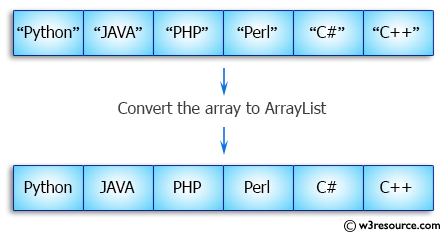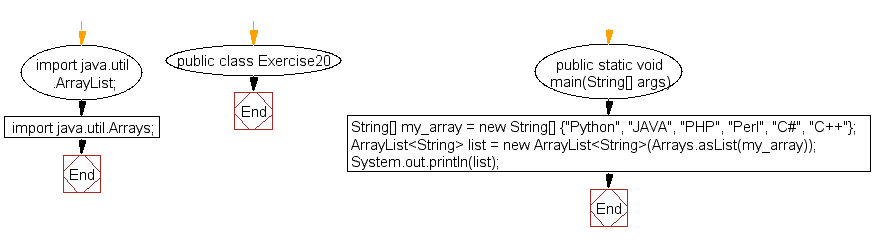﻿ Java exercises: Convert an array to ArrayList - w3resource# Java Array Exercises: Convert an array to ArrayList

## Java Array: Exercise-20 with Solution

Write a Java program to convert an array to ArrayList.

Pictorial Presentation:Sample Solution:

Java Code :

``````import java.util.ArrayList;
import java.util.Arrays;
public class Exercise20 {
public static void  main(String[] args)
{
String[]  my_array = new String[] {"Python", "JAVA", "PHP",  "Perl", "C#", "C++"};
ArrayList<String>  list = new ArrayList<String>(Arrays.asList(my_array));

System.out.println(list);
}
}
```
```

Sample Output:

```[Python, JAVA, PHP, Perl, C#, C++]
```

Flowchart:Visualize Java code execution (Python Tutor):

Java Code Editor:

Improve this sample solution and post your code through Disqus

What is the difficulty level of this exercise?

Test your Programming skills with w3resource's quiz.

﻿

## Java: Tips of the Day

countOccurrences

Counts the occurrences of a value in an array.

Use Arrays.stream().filter().count() to count total number of values that equals the specified value.

```public static long countOccurrences(int[] numbers, int value) {
return Arrays.stream(numbers)
.filter(number -> number == value)
.count();
}
```

Ref: https://bit.ly/3kCAgLb